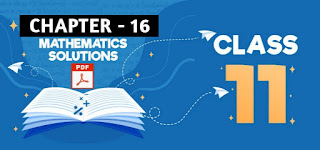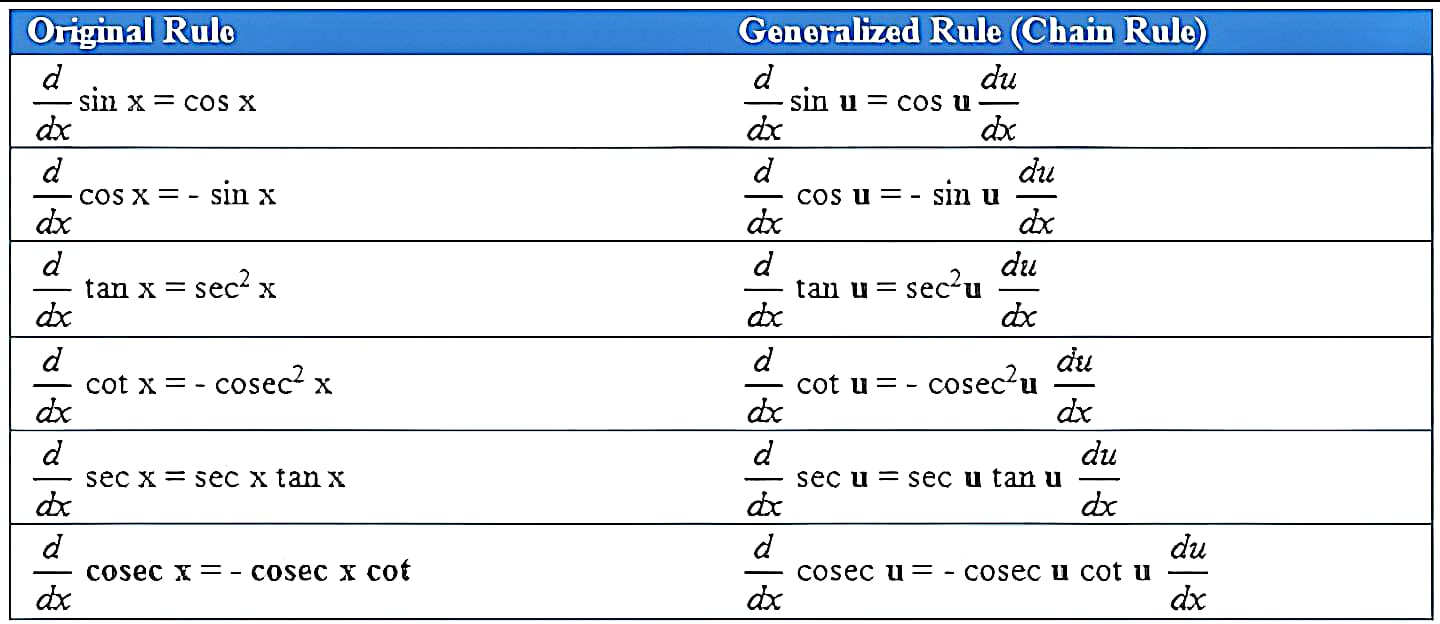# The Derivatives: Class 11 Mathematics Solutions | Exercise - 16.2## Chapter - 16  The Derivatives

In the following chapter, we will learn some fundamental skills that are important to the understanding and application of derivatives. We will learn how to find derivatives of algebraic, trigonometric, exponential, and logarithmic functions both by definition (simple forms) and by differentiation rules.

In order to get a good understanding of derivatives, let us start with a brief overview of some of the subtopics related to derivatives and a few of the important formulas that come along with them.

### Introduction

Differential calculus is a theory which has its origin in the solution of two old problems- one of drawing a tangent line to a curve and the other of calculating the velocity of non-uniform motion of a particle. In both the problems, the curves involved are continuous curves and the process used is the limiting process.

So the objects of study in the differential calculus are continuous functions. These problems were solved in a certain sense by Isaac Newton (English, 1642-1727) and Gottfried Willhelm Leibnitz (German, 1646-1716) and in the process differential calculus is discovered.

### Increment

A small change in the value of a variable is known as the increment. A change in the value of the variable may increase or decrease. But in either case, we use the term increment to denote the change. As for example, if the value of the variable changes from 2.0 to 2.0004 or from 2.0004 to 2.0 the change known as the increment is 0.0004.

Let y = f(x) be the function of x. If the value of x changes, the value of y will also change accordingly. Generally we denote by Δx (read as 'delta x') to change in x and Δy the corresponding change in y.

If x changes to x + Δx, y will change to y + Δy and f(x) changes to f(x + Δx) that is, f(x) = 2x + 3 then f(x + Δx) = 2(x + Δx) + 3

### Derivatives of the Trigonometric Functions

This specific exercise is all about derivatives of trigonometric functions. In the previous exercise we study about the ways of finding derivative of a function using product rule, sum rule, power rule, quotient rule and the chain rule. But in this exercise, we won't talk about those things. However we may need to use some of the formula of previous exercise while solving problems of this exercise too.

Some of the formula for the derivatives of trigonometric functions used in this exercise are given below. Try to remember them so that it will be easy while solving the problems given in the exercise book.### Exercise - 16.2

You will find all the solutions of class 11, the derivatives chapter exercise 16.2, in this PDF file. However, if you are looking for solutions of exercise 16.1 or exercise 16.3, then you need to click the button given above.

NoteScroll the PDF to view all Solution

You are not allowed to post this PDF in any website or social platform without permission.

## Is Class 11 Mathematics Guide Helpful For Student ?

I have published this Notes for helping students who can't solve difficult maths problems. Student should not fully depend on this note for completing all the exercises. If you totally depend on this note and simply copy as it is then it may affect your study.

Student should also use their own will power and try to solve problems themselves. You can use this mathematics guide PDF as a reference. You should check all the answers before copying because all the answers may not be correct. There may be some minor mistakes in the note, please consider those mistakes.

## How to secure good marks in Mathematics ?

As, you may know I'm also a student. Being a student is not so easy. You have to study different subjects simultaneously. From my point of view most of the student are weak in mathematics. You can take me as an example, I am also weak in mathematics. I also face problems while solving mathematics questions .

If you want to secure good marks in mathematics then you should practise them everyday. You should once revise all the exercise which are already taught in class. When you are solving maths problems, start from easy questions that you know already. If you do so then you won't get bored.

Maths is not only about practising, especially in grade 11 you to have the basic concept of the problem. When you get the main concept of the problem then you can easily any problems in which similar concept are applied.

When your teacher tries to make the concept clear by giving examples then all students tries to remember the same example but you should never do that. You can create your own formula which you won't forget later.

If you give proper time for your practise with proper technique then you can definitely score a good marks in your examination.

Disclaimer: This website is made for educational purposes. If you find any content that belongs to you then contact us through the contact form. We will remove that content from our as soon as possible.

If you have any queries then feel free to comment down.
No Comment